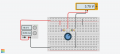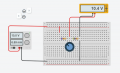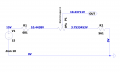# I can't understand how to translate this into math: potentiometer as voltage divider.

#### hederahelix

Joined Jun 19, 2021
2
Teacher gave us this circuit to analyze and we're supposed to use the voltage divider formulation:
$V_{R1} = \frac{E\cdot R1}{R1+R2+R3}$

It gives me:
$V_{R1} = 4.55$
$V_{R1} = 6.69$
$V_{R1} = 3.75$

The problem is, either I don't know how to interpret these numbers, or I'm not using the right logic. I need the Vout for the Pot to be the same as the calculations, but the simulator gives me 3.75 at low and 10.4 at high. I'm really confused, if anyone could explain how I'm supposed to read this data I'd be really thankful!#### ericgibbs

Joined Jan 29, 2010
14,114
hi hedera,
Welcome to AAC,
I see that you have used LTSpice.? Please post your LTS asc file.

Have you been given the value of R1, R2 and R3.?
E

#### LesJones

Joined Jan 8, 2017
3,570
First thing is you don't seem to be able to read the resistor values correctly. You need to understand the resistor colour code. (Note in this case you miss reading of the resistor colour code is only giving you a very small error.)

How did you obtain the track resistance of the potentiometer ? (You don't seem to have been given itdirectly in the initial information but you have been given enough information to calculate it.)

Les.

#### hederahelix

Joined Jun 19, 2021
2
The values of the resistors R1 and R3 were given to me and it is the exact input on both simulators: 681 and 561, also the value for 1k on the potentiometer.

Les, I don't know what you mean by track resistance in this contextThe .asc file, as requested>

#### Attachments

• 892 bytes Views: 3

#### crutschow

Joined Mar 14, 2008
28,155
Your formula does not match the schematic.

•Deleted member 115935

#### LesJones

Joined Jan 8, 2017
3,570
The track of the potentiometer is the resistive part that terminates at the two outside connections. The middle connection goes to the wiper which makes contact with the track as the shaft is turned the point of contact between the wiper and track moves from one end to the other. If your teacher gave you those values for the resistors then he is wrong. He has not interpreted the third coulor as a decimal multiplyer. For example the right hand resistor green, blue, brown, gold. Green is 5, blue is 6 and brown is one. But its value is not 561 it is 56 x 10^1. 10 to the power 1 is 10 so this makes the value 56 x 10 = 560. The last colour gold is the tolerance which is 5% so the any sample of the resistor will be 560 ohms + or - 5%. So you can see this in this case the error in reading the value has made very little difference. If the third colour had been yellow (4) then the error would have been 1000 times. The teacher would have said it was 564 ohms when it was really 560000 ohms. You have not shown how you have put values into the formula so I don't know where you went wrong. I don't see how you can have 3 answers when the pictures only show two conditions. (With the wiper positioned at each end of the track.)

Les.

Last edited:

#### Ramussons

Joined May 3, 2013
1,098
Vr1 = 4.55 V
Vpot = 6.69 V
Vr2 = 3.75 V

#### crutschow

Joined Mar 14, 2008
28,155
An alternate way to calculate the divider voltage (which I find easier) is to first calculate the current through the three resistors, and then use that current to calculate the IR drop through the resistance to ground from the bottom resistance plus the pot resistance to the wiper location.

•xox

#### MrAl

Joined Jun 17, 2014
8,543
Teacher gave us this circuit to analyze and we're supposed to use the voltage divider formulation:
$V_{R1} = \frac{E\cdot R1}{R1+R2+R3}$

It gives me:
$V_{R1} = 4.55$
$V_{R1} = 6.69$
$V_{R1} = 3.75$

The problem is, either I don't know how to interpret these numbers, or I'm not using the right logic. I need the Vout for the Pot to be the same as the calculations, but the simulator gives me 3.75 at low and 10.4 at high. I'm really confused, if anyone could explain how I'm supposed to read this data I'd be really thankful!

View attachment 241722
View attachment 241723
View attachment 241724
Hello,

When you adjust the pot the output voltage changes, therefore the nomenclature "VR1" in not correct. "VR1" in circuit analysis would mean "The voltage across R1", but you seem to be calculating the pot 'arm or wiper' voltage, we might call Vw. That is a different kind of calculation and that means that the formula resistor values R1, R2, R3 do not correlate to the circuit values R1 and R2 although there is a more profound reason why they dont correlate. The formula R's are just the formula R's, while the circuit R's are the circuit R's. They are not the same, and this is quite typical because we often use formulas with predefined variables and we have to figure out what the value of those variables are IN THE FORMULA as they correlate to our circuit in hand.

So your first job is to identify the values in the FORMULA from the values in the CIRCUIT.
Forget for a minute the variables in the formula are R1, R2, R3, and instead change them to:
Ra, Rb, Rc
and i think this will start to make a LOT more senseAnother quick example using Ohm's Law:
V=I*R

Now if we have a circuit and make measurements, does the resistor on the PC board have to be labeled "R"? No, not at all. It will be maybe R1, R2, or R105, but in the formula it becomes just plain "R".

#### MrChips

Joined Oct 2, 2009
24,593
Ok. I reread your problem and my conclusion matches what other members have posted.

1) R1 = 680Ω and R2 = 560Ω although 1Ω discrepancy will hardly make any difference.
2) The only formula you need to solve this is Ohm's Law.
3) The only discrepancy I can observe is why there are two different values shown, 10.4438V and 10.43711V. Otherwise the numbers are close enough to what is expected.

#### Wolframore

Joined Jan 21, 2019
2,373
Ok. I reread your problem and my conclusion matches what other members have posted.

1) R1 = 680Ω and R2 = 560Ω although 1Ω discrepancy will hardly make any difference.
2) The only formula you need to solve this is Ohm's Law.
3) The only discrepancy I can observe is why there are two different values shown, 10.4438V and 10.43711V. Otherwise the numbers are close enough to what is expected.
R1 is 681 and R2 is 581
also it seems that the pot in LTSpice is at 1 not 0. Which would indicate 990 and 10